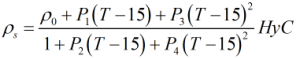# C255 – ASTM-IP Table 53

## Description

This is a density referral calculation that replicates ASTM IP Petroleum Measurement Table – Table 53.

ASTM-IP Petroleum Measurement Tables (1953) – Reprinted 1974

Kelton calculation reference C255

FLOCALC calculation reference F062

## Options

### Hydrometer Correction

Check box depending on what whether you wish to apply the hydrometer correction to the observed density.

## Calculation

### Density calculation

The standard density calculated using an iterative regime centred around the equation:Where ρ0 = Initial density T = Temperature Pn = Parameter based upon derivatives relating the change in density to the change in temperature HyC = Hydrometer Correction

Back